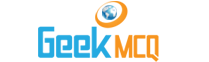# Entry Test MCQ :: Road Problems

1.  If you travel 120 Kms in 3 hours then your speed of traveling per hour will be :
 A. 20 B. 80 C. 40 D. 100

2.  A man walked 3300 meters in 30 minutes. His speed for journey in meters per minute will be:
 A. 120 B. 125 C. 100 D. 110

3.  A boy is traveling along a highway at a uniform speed of 50 km/h. What will be the distance traveled in 1
 A. 35 B. 65 C. 95 D. 75

4.  An athlete runs 1 km in 3 minutes. The speed of running in meters per minute is
 A. 111? B. 222? C. 444? D. 333?

5.  If I walk at 3 km/h I miss train by 2 minutes. If however I walk at 4 km km/h I reach the station 3 minutes earlier. What is the distance of station?
 A. 3/4 km B. 4/5 km C. 1 km D. 5/4 km

6.  A motorist travels to a place 100 Kms away at an average speed of 50 km/h and returns with 40 km/h. His average speed for the whole journey is:
 A. 44.4 km/h B. 45 km/h C. 45.4 km/h D. 48 km/h

7.  If I walk to my office at 6 km/h I arrive 6 minutes earlier. If however I walk at 4 km/h I arrive 4 minute late. The distance I have to travel is:
 A. 2 B. 2.5 C. 3 D. 4

8.  A and B cycle from Lahore to Kasur a distance of 192 km/h at 18 and 14 km/h simultaneously. A reaches Kasur and starts back to Lahore. How for from Kasur will he meet B?
 A. 12 km B. 16 km C. 24 km D. 42 km

9.  In a race of one km A beats B by 5 seconds and by a distance of 40 meters. How long does B take to run one km.
 A. 120 seconds B. 125 seconds C. 130 seconds D. 150 seconds

10.  A motorcyclist goes from Karachi to Hyderabad a distance of 192 km at an average speed of 32 km/h. Another man driving a car starts 2 1/2 hours after the first but reaches there half an hour earlier. The speed of car is:
 A. 64 B. 96 C. 80 D. 25.6